# How to Draw a Clock

Let's try to visualise the data points

The goal of this notebook is to visualise the data points we have. This is definitely not an exact representation and makes a lot of simpilying assumtions. All comments and improvement suggestions are welcome in the comments! For the end result just scroll right to the bottom

In :
import numpy as np
import pandas as pd
import matplotlib.pyplot as plt

In :
train = pd.read_csv('../data/train.csv', index_col='row_id')

In :
example = train.loc['XX9B1M32E548Z42HB']


# Setup¶

We are going to use polar coordinates. These are defined by a radius r and an angle theta:

In :
fig, ax = plt.subplots(
figsize=(4,4),
subplot_kw={'projection': 'polar'}
)
ax.set_rmax(1)

for i in range(1,13):
theta = np.pi / 2 - i*np.deg2rad(360/12)
r = 0.7
plt.plot([theta,0], [r, 0], color='red')

ax.text(
theta, r, str(i),
ha='center',
va='center',
fontsize=22
)

plt.show()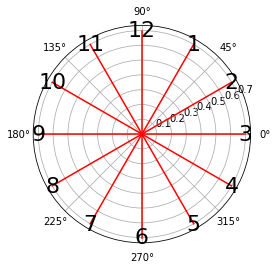Now let's try to take the image closer to the data

# Digits¶

For digits, we need to define two things: position and relative size.

## Position¶

We don't have exact digit positions in the data, but using {i} dist from cen (distance from the centre) and euc_dist_digit_{i} (i.e. distance from a corresponding red line above) we can find the angle between the actual digit position and the red line from the right-angle triangle formed by all these lines. Hence we still don't know whether the angle is clockwise or counterclockwise, we'll assume it's always ccw

In :
def digit_coordinates(i, example):
dist_from_centre = example[f'{i} dist from cen']
if not dist_from_centre:
return None, None

# calculate the angle
sin_angle_deviation = example[f'euc_dist_digit_{i}'] / dist_from_centre
theta = np.pi / 2 - i*np.deg2rad(360/12) + np.arcsin(sin_angle_deviation)
return theta, dist_from_centre

In :
# we plot on a grid with radius of 1 and input images are 1024x1024 so we scale everything by 512
scale_coef = 512
fontsize = 22

In :
fig, ax = plt.subplots(
figsize=(4,4),
subplot_kw={'projection': 'polar'}
)

ax.set_rmax(1)

# generate blank canvas
ax.set_rticks([])
ax.set_thetagrids([])
ax.grid(False)
# ax.spines['polar'].set_visible(False)

# plot digits
for i in range(1, 13):
theta, d = digit_coordinates(i, example)
fontsize = 22
if not pd.isna(d):
r = d / scale_coef
plt.plot([theta,0], [r, 0], color='red')
ax.text(
theta, r, str(i),
ha='center',
va='center',
fontsize=fontsize
)

plt.show()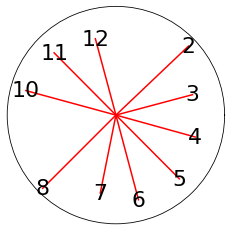## Size¶

We have height and width of the digits. We will use width to scale the fontsize and ignore height, for simplicity

In :
font_scale = 270 / 2 # figure size (4,4) produces ~270x270 images
# so we another scaler to match pixel font size

In :
fig, ax = plt.subplots(
figsize=(4,4),
subplot_kw={'projection': 'polar'}
)

ax.set_rmax(1)

# generate blank canvas
ax.set_rticks([])
ax.set_thetagrids([])
ax.grid(False)
# ax.spines['polar'].set_visible(False)

# plot digits
for i in range(1, 13):
theta, d = digit_coordinates(i, example)
if not pd.isna(d):
r = d / scale_coef
plt.plot([theta,0], [r, 0], color='red')

try:
width = example[f'width_digit_{i}'] / scale_coef
fontsize = round(width * font_scale)
except ValueError:
pass

ax.text(
theta, r, str(i),
ha='center',
va='center',
fontsize=fontsize
)

plt.show()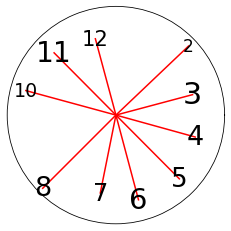# Hands¶

For hands we will use minute_pointing_digit and hour_pointing_digit since we already know the digit coordinates, and we have lengths in the data

In :
fig, ax = plt.subplots(
figsize=(4,4),
subplot_kw={'projection': 'polar'}
)

ax.set_rmax(1)

# generate blank canvas
ax.set_rticks([])
ax.set_thetagrids([])
ax.grid(False)
ax.spines['polar'].set_visible(False)
ax.autoscale(False)

# plot digits
for i in range(1, 13):
theta, d = digit_coordinates(i, example)
if not pd.isna(d):
r = d / scale_coef

width = example[f'width_digit_{i}'] / scale_coef
fontsize = round(width * font_scale)

ax.text(
theta, r, str(i),
ha='center',
va='center',
fontsize=fontsize
)

if example['minute_pointing_digit'] == i:
m = example['minute_hand_length'] / scale_coef
plt.plot([theta,0], [m, 0], linewidth=3)

if example['hour_pointing_digit'] == i:
m = example['hour_hand_length'] / scale_coef
plt.plot([0,theta], [0, m])
plt.show()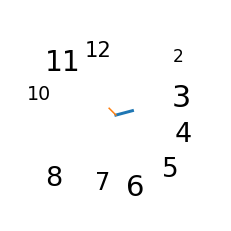Almost done! We can make hands position a bit more realistic using deviation_from_centre - how far the implied centre is from (0,0) and intersection_pos_rel_centre to pick the right quadrant (we'll assume the point is always the middle of it). See complete code below.

# Putting it all together¶

In :
centre_theta_dict = {
'BR': np.pi/4,
'BL': 3*np.pi/4,
'TL': 5*np.pi/4,
'TR': 7*np.pi/4,
}

def draw_a_clock(example, ax):
ax.set_rmax(1)

# generate blank canvas
ax.set_rticks([])
ax.set_thetagrids([])
ax.grid(False)
ax.spines['polar'].set_visible(False)
ax.autoscale(False)

try:
centre_r = example['deviation_from_centre'] / scale_coef
centre_theta = centre_theta_dict[example['intersection_pos_rel_centre']]
except (ValueError, KeyError):
centre_r, centre_theta = 0, 0

# plot digits
for i in range(1, 13):
theta, d = digit_coordinates(i, example)
if not pd.isna(d):
r = d / scale_coef

width = example[f'width_digit_{i}'] / scale_coef
fontsize = round(width * font_scale)

ax.text(
theta, r, str(i),
ha='center',
va='center',
fontsize=fontsize
)

if example['minute_pointing_digit'] == i:
m = example['minute_hand_length'] / scale_coef
ax.plot([theta,centre_theta], [m, centre_r], linewidth=2)

if example['hour_pointing_digit'] == i:
m = example['hour_hand_length'] / scale_coef
ax.plot([centre_theta,theta], [centre_r, m], linewidth=3)
ax.set_title(f"{example['diagnosis'].replace('_', '-').capitalize()}", va='top', ha='center')

In :
row, col = 3, 2

c = 'normal'

fig, axes = plt.subplots(row, col,
figsize=(4*col,4*row),
subplot_kw={'projection': 'polar'}
)

for ax in axes.reshape(-1):
example = train[train['diagnosis'] == c].sample(1).iloc
draw_a_clock(example, ax)

plt.show()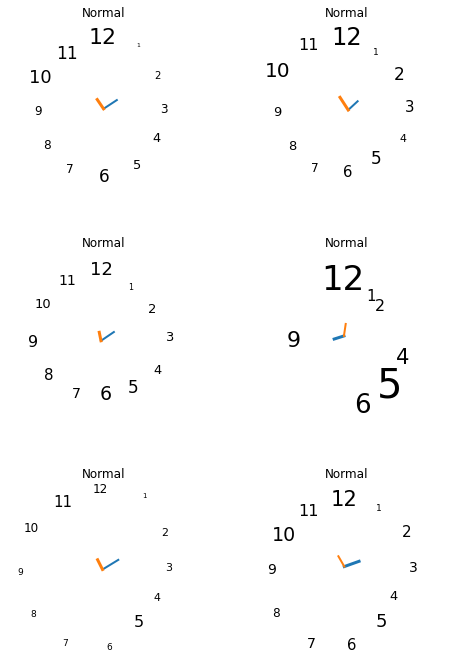In :
row, col = 3, 2

c = 'post_alzheimer'

fig, axes = plt.subplots(row, col,
figsize=(4*col,4*row),
subplot_kw={'projection': 'polar'}
)

for ax in axes.reshape(-1):
example = train[train['diagnosis'] == c].sample(1).iloc
draw_a_clock(example, ax)

plt.show()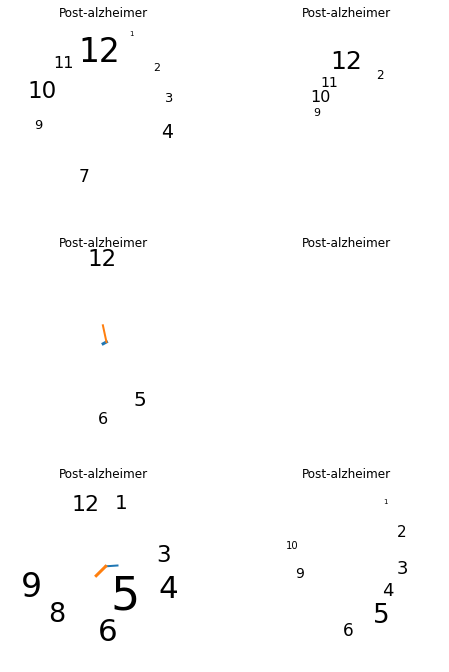In :
row, col = 3, 2

c = 'pre_alzheimer'

fig, axes = plt.subplots(row, col,
figsize=(4*col,4*row),
subplot_kw={'projection': 'polar'}
)

for ax in axes.reshape(-1):
example = train[train['diagnosis'] == c].sample(1).iloc
draw_a_clock(example, ax)

plt.show()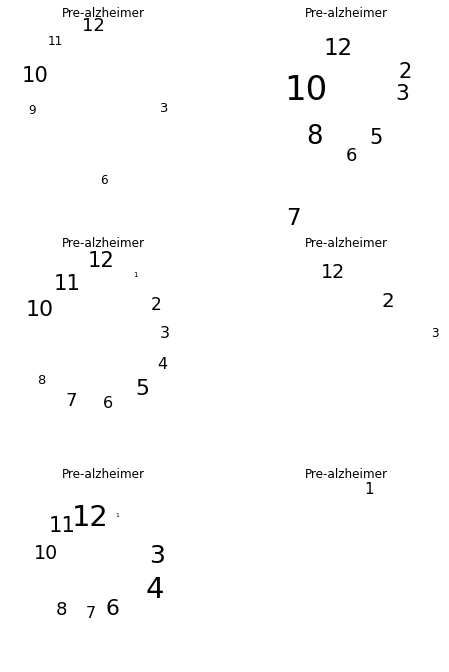1470santiactis
Almost 2 years ago

This is awesome! It’s really interesting to see the data transformed into actual images of what someone might have drawn.AliGebily
Almost 2 years ago

Great JobJohnowhitaker
Almost 2 years ago

Great notebook! As @By santiactis said, cool to see hints about what the drawings might have looked at. This is much neater than the code I used for my video, which I was too embarrassed to share :) Did you play with ‘ellipse_circle_ratio’ or the ‘between_digits’ columns? I thought it was a bit of a quirky feature - some people draw all the digits close together but presumably because of the code used by the folks preparing data for the contest the angle sum includes all pairs of digits, meaning it is almost always 360 degrees! The variance (eg ‘between_digits_angle_cw_var’) does have quite a spread - I used it to add some randomness to the angles even though there isn’t a way to actually predict what this looked like.  - https://www.youtube.com/watch?v=xByX4G1gK-M